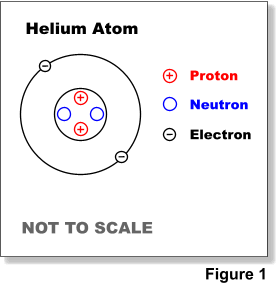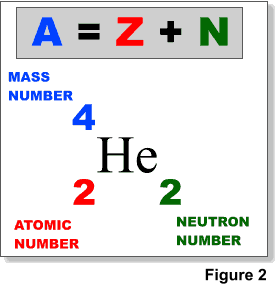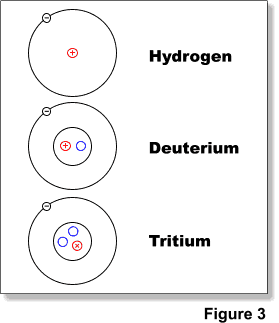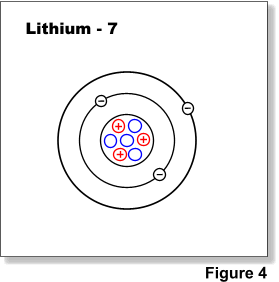#### Atoms are the extremely small particles that are the basic building blocks of ordinary matter.

Atoms can join together to form molecules, which make up most objects. Different elements (e.g. oxygen, carbon, uranium) are made up of different types of atoms. An atom is the smallest unit of an element that will behave as that element.

Atoms consist of an extremely small, positively charged nucleus surrounded by a cloud of negatively charged electrons. Although typically the nucleus is less than one ten-thousandth the size of the atom, the nucleus contains more that 99.9% of the mass of the atom. Nuclei are made of positively charged protons and electrically neutral neutrons held together by a nuclear force. This force is much stronger than the electrostatic force that binds electrons to the nucleus, but its range is limited to distances of the order of 1 x 10-15 meters. See Figure 1.The number of protons in the nucleus is called the atomic number (Z), the atomic number defines the element. The number of neutrons in the nucleus is denoted by N. The mass number (A) of the nucleus is equal to Z + N (Figure 2). The mass of the nucleus in atomic mass units (amu) is usually slightly different from the mass number.

Atoms of the same element can have different number of neutrons and they are called isotopes of that element. As an example hydrogen has three (3) isotopes: hydrogen-1 (hydrogen), hydrogen-2 (deuterium) and hydrogen-3 (tritium) (Figure 3).

At present there are 118 known elements which are typically displayed on the periodic table of the elements. Elements with atomic numbers 1 – 98 have all been shown to exist in nature while elements with atomic number 99 – 118 have only ever been produced artificially.

Nuclides are often identified using the name of the element and the mass number (total number of protons and neutrons), i.e. lithiu-7, uranium-238, etc. (Figure 4). When referring to nuclides of the same element they are generally term isotopes i.e. uranium-238 & uranium-235. Radioactive nuclides or isotopes are called radionuclides or radioisotopes.

Among the elements there are 253 stable nuclides, and more than 3000 radioisotopes. The majority of the radioisotopes (>2400) are artificially produced and not presently found in nature.